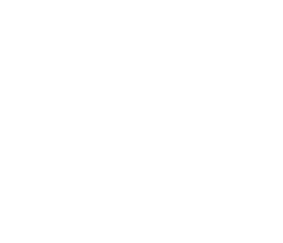# Maths MCQs for Class 12 with Answers Chapter 6 Application of Derivatives

Free PDF Download of CBSE Maths Multiple Choice Questions for Class 12 with Answers Chapter 6 Application of Derivatives. Maths MCQs for Class 12 Chapter Wise with Answers PDF Download was Prepared Based on Latest Exam Pattern. Students can solve NCERT Class 12 Maths Application of Derivatives MCQs Pdf with Answers to know their preparation level.

## Application of Derivatives Class 12 Maths MCQs Pdf

1. The total revenue in ₹ received from the sale of x units of an article is given by R(x) = 3x² + 36x + 5. The marginal revenue when x = 15 is (in ₹ )
(a) 126
(b) 116
(c) 96
(d) 90

Explaination:
(a), as R'(x) = 6x + 36
⇒ R'(15) = 90 + 36 = 126

2. The side of an equilateral triangle is increasing at the rate of 2 cm/s. The rate at which area increases when the side is 10 is
(a) 10 cm²/s
(b) √3 cm²/s
(c) 10√3 cm²/s
(d) $$\frac{10}{3}$$cm²/s

Explaination: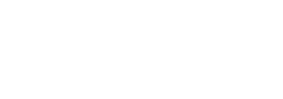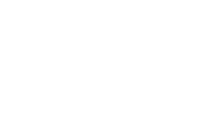3. The point(s) on the curve y = x², at which y-coordinate is changing six times as fast as x-coordinate is/are
(a) (2, 4)
(b) (3, 9)
(c) (3, 9), (9, 3)
(d) (6, 2)

Explaination: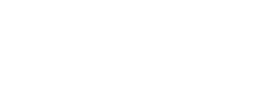4. The equation of the normal to the curve y = sin x at (0, 0) is
(a) x = 0
(b) y = 0
(c) x + y = 0
(d) x – y = 0

Explaination: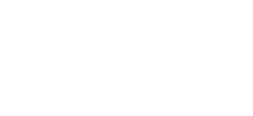5. The point on the curve where tangent to the curve y2 = x, makes an angle of 45° clockwise with the x-axis is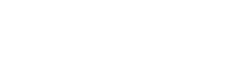Explaination: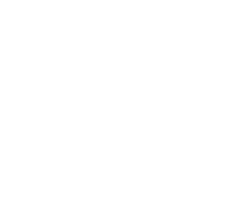6. The line y = x + 1 is a tangent to the curve y2 = 4x at the point
(a) (-1, 2)
(b) (1, 2)
(c) (1, -2)
(d) (2, 1)

Explaination:
(b), as slope of line y = x + 1 is 1
and $$\frac{dy}{dx}$$ = $$\frac{2}{y}$$ = 1
⇒ y = 2 and x = 1.
Therefore point is (1, 2).

7. The curves y = ae-x and y = bex are orthogonal if
(a) a = b
(b) a = -b
(c) ab = -1
(d) ab = 1

Explaination: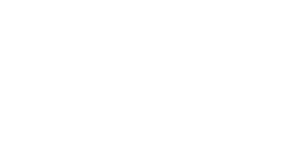8. If the curves ay + x2 = 7 and x3 = y cut orthogonally at (1,1), then the value of a is
(a) 1
(b) 0
(c) -6
(d) 6

Explaination: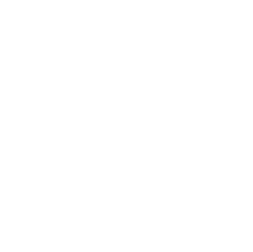9. The tangent to the curve y = e2x at the point (0, 1) meets the x-axis at
(a) (0, 1)
(b) (2, 0)
(c) (-$$\frac{1}{2}$$, 0)
(d) (-2, 0)

Explaination: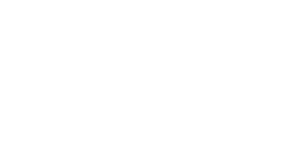10. The angle between the curve y² = x and x² =y at (1, 1) is
(a) 60°
(b) tan-1$$\frac{4}{3}$$
(c) cot-1$$\frac{4}{3}$$
(d) 90°

Explaination: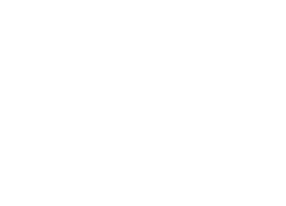11. The absolute maximum value of y = x3 – 3x + 2 in 0 ≤ x ≤ 2 is
(a) 4
(b) 6
(c) 2
(d) 0

Explaination:
(a), as y’ = 3x² – 3, for a point of absolute maximum or minimum y’=0 ⇒ x = ± 1.
y]x=0 = 2,
y]x=1 = 1 – 3 + 2 = 0,
y]x=-1 = -1 +3+ 2 = 4,
y]x=2 = 8 – 6 + 2 = 4

12. Diameter of a sphere is $$\frac{3}{2}$$(2x + 5), the rate of change of its surface area with respect to x is ____ .

Explaination: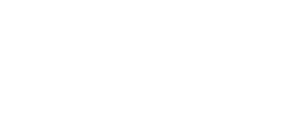13. The edge of a cube is increasing at the rate of 0.3 cm/s, the rate of change of its surface area when edge is 3 cm is ____ .

Explaination: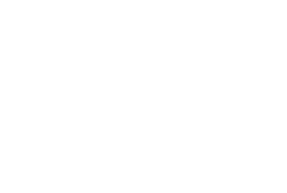14. The rate of change of area of a circle with respect to its radius is _______ .

Explaination:
2πr, as A = πr²
⇒ $$\frac{dA}{dr}$$ = 2πr

15. Radius of a variable circle is changing at the rate of 5 cm/s. What is the radius of the circle at a time when its area is changing at the rate of 100 cm²/s? [HOTS]

Explaination: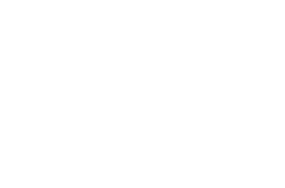16. Find the point on the curve y=x², where the rate of change of x-coordinate is equal to the rate of change of y-coordinate.

Explaination: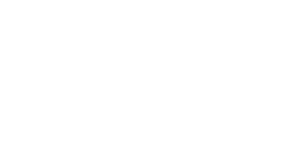17. The side of an equilateral triangle is increasing at the rate of 0.5 cm/s. Find the rate of increase of its perimeter.

Explaination: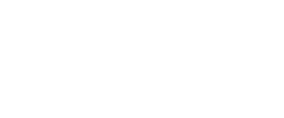18. If the rate of change of volume of a sphere is equal to the rate of change of its radius, then find the radius.

Explaination: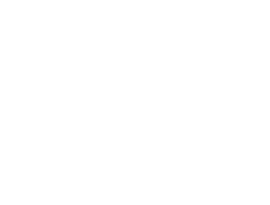19. The volume of a sphere is increasing at the rate of 3 cubic centimeter per second. Find the rate of increase of its surface area, when the radius is 2 cm. [Delhi 2017]

Explaination: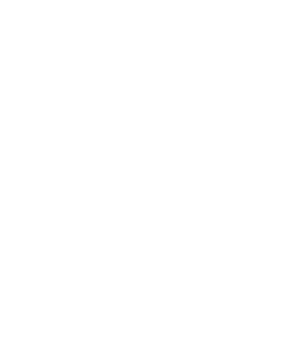20. For the curve y = 5x – 2x3, if x increases at the rate of 2 units/s, then find the rate of change of the slope of the curve when x = 3. [Delhi 2017]

Explaination: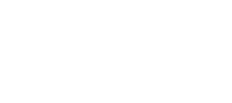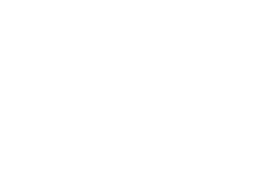21. The volume of a cube is increasing at the rate of 9 cm3/s. How fast is its surface area increasing when the length of an edge is 10 cm? [AI 2017]

Explaination: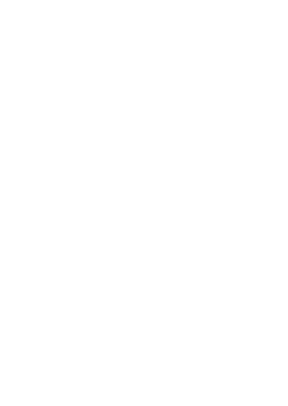22. The volume of a sphere is increasing at the rate of 8 cm3/s. Find the rate at which its surface area is increasing when the radius of the sphere is 12 cm. [AI 2017]

Explaination: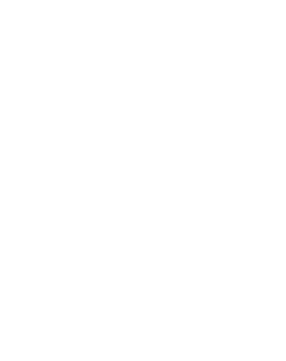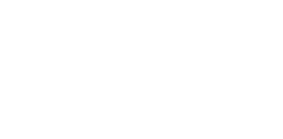23. The radius r of a right circular cylinder is decreasing at the rate of 3 cm/min. and its height h is increasing at the rate of 2 cm/ min. When r = 7 cm and h = 2 cm, find the rate of change of the volume of cylinder.
[Use π = $$\frac{22}{7}$$] [Foreign 2017]

Explaination: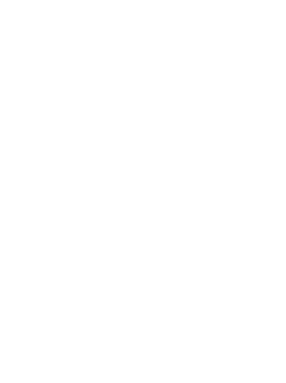24. The radius r of the base of a right circular cone is decreasing at the rate of 2 cm/min and its height h is increasing at the rate of 3 cm/min. When r = 3.5 cm and h = 6 cm, find the rate of change of the volume of the cone. [Use π = $$\frac{22}{7}$$]

Explaination: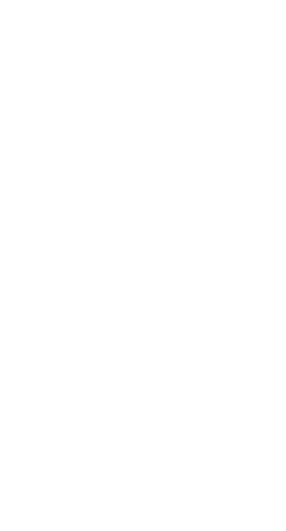25. The function f(x) = 4x + 3, x ∈ R is an increasing function. State true or false.

Explaination: True, as f'(x) = 4 > 0. Hence, increasing.

26. The function f(x) = log(cos x) is increasing function for [0, $$\frac{\pi}{2}$$] State true or false.

Explaination: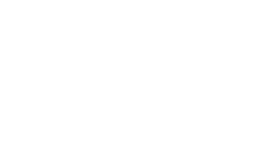27. Show that function y = 4x – 9 is increasing for all x ∈ R.

Explaination:
Given y = 4x – 9
$$\frac{dy}{dx}$$= 4 > 0 for all x ∈ R.
Hence, function is increasing for all x ∈ R.

28. Show that the function given by f(x) = sin x is strictly decreasing in ($$\frac{\pi}{2}$$, π).

Explaination:
Consider f(x) = sin x
f(x) = cos x …(i)
cos x < 0 for each x ∈ ($$\frac{\pi}{2}$$, π)
∴ f(x) < 0 [from (i)]
Hence, function is strictly decreasing in ($$\frac{\pi}{2}$$, π).

29. Show that the function y = $$\frac{3}{x}$$ + 7 is strictly decreasing for x ∈ R (x ≠ 0).

Explaination:
y’= $$\frac{3}{x²}$$ < 0, for x ∈ R, x ≠ 0.
Hence, function is strictly decreasing.

30. Show that the function f(x) = log |cos x| is strictly decreasing in (0, $$\frac{\pi}{2}$$) [HOTS]

Explaination:
f'(x)= $$\frac{1}{\cos x}$$.(-sinx) = -tan x., tan
x > 0 for (0, $$\frac{\pi}{2}$$) f'(x) < 0
Hence, function is strictly decreasing.

31. Prove that the function given by f(x) = x3 – 3x² + 3x – 100 is increasing in R. [NCERT]

Explaination:
f'(x) = 3x² – 6x + 3 = 3(x² – 2x + 1)
= 3(x – 1)² > 0. Hence, f is increasing in R.

32. Find the interval for which the function f(x) = cot-1 x + x increases.

Explaination: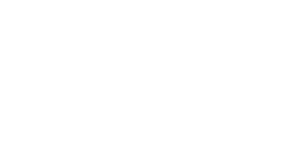33. ShoW that the function fix) = 4×3 – 18X2 + 27x – 7 is always increasing on R. [Delhi 2017]

Explaination: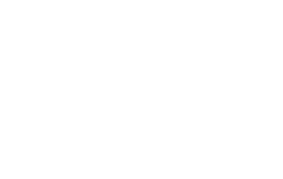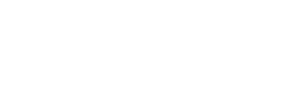34. Show that the function f given by f(x)=tan-1(sinx+cos x) is decreasing for all ($$\frac{\pi}{4}$$, $$\frac{\pi}{2}$$). [Foreign 2017]

Explaination: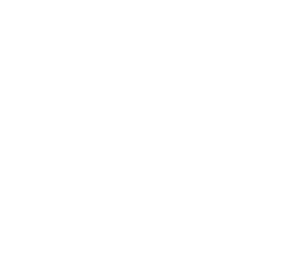35. Tangent to the curve given by x = sec θ and y = cosec θ, at θ = $$\frac{\pi}{2}$$ makes an angle _______ with the x-axis.

Explaination: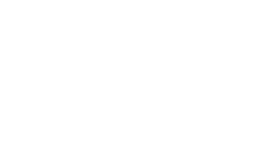36. Prove that the tangents to the curve y = x3 + 6 at the points (-1, 5) and (1, 7) are parallel. [HOTS]

Explaination:
y = x3 + 6
⇒ y’ = 3x²
y’](-1, 5) = 3(-1)² = 3 and y’](1, 7)
= 3(1)² = 3
As slope at these points are equal. Hence, tangents are parallel.

37. At what point on the curve y = x² does the tangent make an angle of 45° with the x-axis? [HOTS]

Explaination:
Slope of the tangent = tan 45° = 1.
y’ = 2x
⇒ 2x = 1
⇒ x = $$\frac{1}{2}$$. Substituting in curve, we get
y = $$\frac{1}{4}$$. Point is ($$\frac{1}{2}$$. $$\frac{1}{4}$$).

38. Find the slope of the tangent to the curve x = 3t² + 1, y = t3 – 1 at x = 1.

Explaination: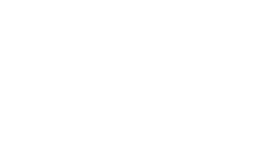39. If the radius of a sphere is measured as 9 cm with an error of 0.03 cm, then find the approximate error in calculating its

Explaination:
Let r be the radius of the sphere and Δr be the error in measuring the radius.
Then r = 9, Δr = 0.03, Surface area of a sphere is given by S = 4πr²
ΔS = $$\frac{dS}{dr}$$.Δr = 8πr.Δr = 8π × 9 × 0.03
= 2.16 π cm²

40. If radius of a circle is increased from 5 cm to 5.1 cm. Find the approximate increase in area.

Explaination:
Area of a circle of radius r is given by, A = πr²
Then, r = 5, r + Δr = 5.1, Δr = 0.1
ΔA =$$\frac{dA}{dr}$$ Δr 2πr × 0.1
= 2π × 5 × 0.1 = π cm²

41. If f(x) = $$\frac{1}{4 x^{2}+2 x+1}$$, then its maximum value is _____ .

Explaination: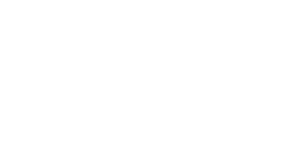42. Show that y = ex has no local maxima or local minima. [HOTS]

Explaination:
y’ ≠ 0, for any x, so no solution of y’ = 0.
Hence, no local maximum or local minimum.

43. It is given that at x = 1, the function f(x)=x4 – 62x² + ax + 9 attains its maximum value on the interval [0,2], Find the value of a. [NCERT]

Explaination:
f'(x)=4x3 – 124x + a, for a point of maximum
f'(1) = 0 ⇒ 4 – 124 + a = 0
⇒ a = 120

44. Find the maximum and minimum values if any of the function given by f(x) = -(x – 1)² + 10. [NCERT]

Explaination:
-(x – 1)² < 0 for x ∈ R
⇒ -(x – 1)²+ 10 ≤ 10
⇒ f(x) ≤ 10,
Maximum value = 10.
Minimum value = nil.

45. Find the maximum and minimum values if any of the function given by f(x) = sin 2x + 5. [NCERT]

Explaination:
-1 ≤ sin 2x ≤ 1
⇒ -1 + 5 ≤ sin 2x+5 ≤ 1 + 5
⇒ 4 ≤ sin 2x + 5 ≤ 6.
Maximum value = 6, Minimum value = 4.

46. Prove that the function f(x)= x3 + x² + x + 1 does not have a maxima or minima.

Explaination:
f'(x) = 3x² + 2x + 1,
f'(x) = 0
⇒ 3x² + 2x + 1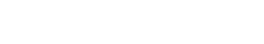As f'(x) ≠ 0 for any real x.
Hence, no maxima or minima.

47. Find the maximum and minimum values, if any, of the function given by f(x) = |sin 4x + 3| [NCERT]

Explaination:
f(x) = |sin 4x + 3|
-1 ≤ sin 4x ≤ 1
⇒ 2 ≤ sin 4x + 3 ≤ 4
⇒ 2 ≤ |sin 4x + 3| ≤ 4.
Minimum value = 2, Maximum value = 4.

48. Find the maximum and minimum value of the function y = |x – 3| + 7, x ∈ R.

Explaination:
∀ x ∈ R
|x – 3| ≥ 0
⇒ |x – 3| + 7 ≥ 7
⇒ y ≥ 7
⇒ minimum value = 7, no maximum value.

49. Find the number which exceeds its square by the greatest possible number.

Explaination:
Let number x exceeds its square by the greatest possible number
y = x – x²
y’ = 1 – 2x,
For maxima y, y’ = 0
⇒ 1 – 2x = 0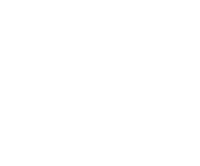50. Given the function f(x) = xx, x > 0, find the stationary point for the function f.